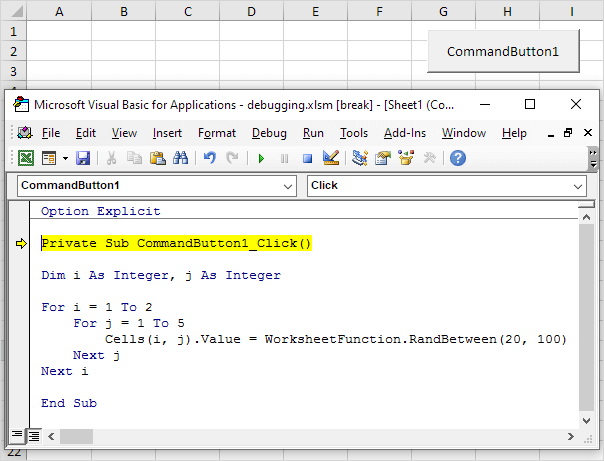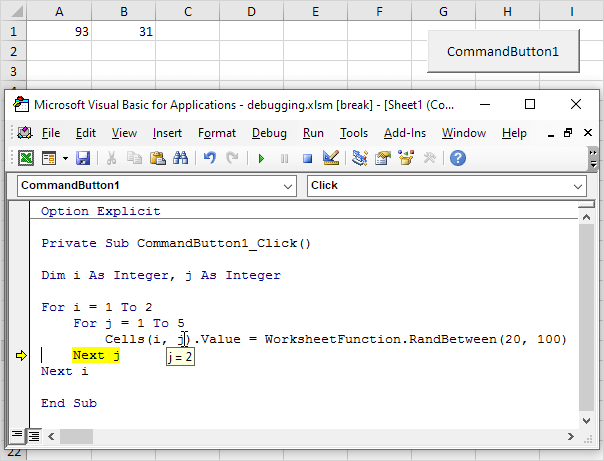# Debugging

This example teaches you how to debug code in Excel VBA.

### Single Step

By pressing F8, you can single step through your code. The is very useful because it allows you to see the effect of each code line on your worksheet.

Place a command button on your worksheet and add the following code lines:

Dim i As Integer, j As Integer

For i = 1 To 2
For j = 1 To 5
Cells(i, j).Value = WorksheetFunction.RandBetween(20, 100)
Next j
Next i

Result when you click the command button on the sheet:1. Empty the range A1:E2.

2. Open the Visual Basic Editor and reduce the size of the screen so that you can see the Visual Basic Editor and worksheet at the same time.

3. In the Visual Basic Editor, place your cursor before Private and press F8.

The first line turns yellow.4. Press F8 four times. For i = 1 and j = 1, Excel VBA enters a random number between 20 and 100 into the cell at the intersection of row 1 and column 1. By holding the cursor steady on a variable, you can see the value of the variable.5. Press F8 two more times. For i = 1 and j = 2, Excel VBA enters a random number between 20 and 100 into the cell at the intersection of row 1 and column 2.6. Single step through the rest of the code to see how Excel VBA enters the other numbers. This is an excellent way to learn how a loop works. If you wish to stop the program, click the Reset (Stop) button.

### Breakpoint

You set a breakpoint to halt execution at a specific code line.

1. Empty the range A1:E2.

2. To set a breakpoint, click on the left margin (in grey) where you want to place the breakpoint. A red dot appears.3. Click on the green arrow to execute the macro until the breakpoint.

Result:Only part of the macro (for i = 1) has been executed.

4. To remove the breakpoint, click on the red dot. Next, click on the green arrow to continue execution.

If you receive a macro from someone else, remember, debugging is great way to understand the code. You can easily see the effect of each code line on your worksheet.

Go to Next Chapter: String Manipulation